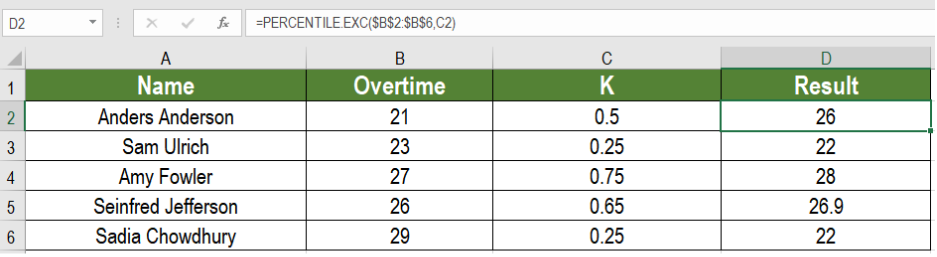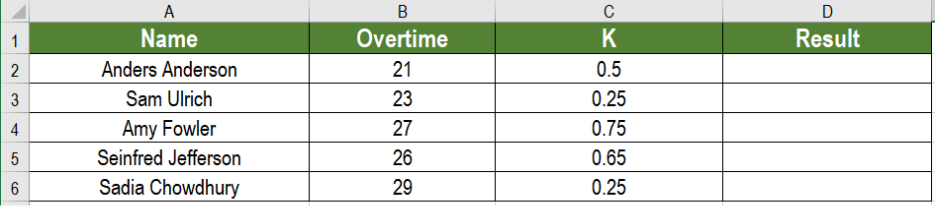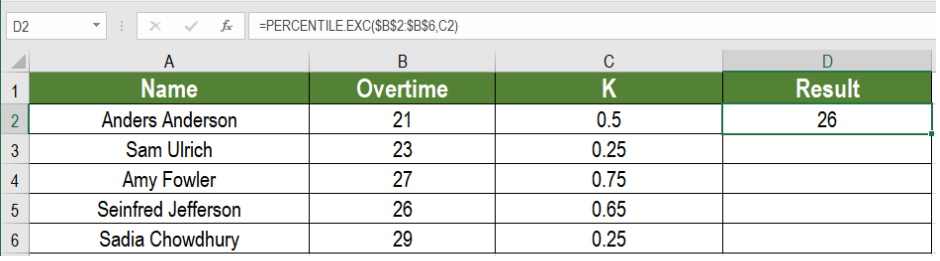Get instant live expert help with Excel or Google Sheets“My Excelchat expert helped me in less than 20 minutes, saving me what would have been 5 hours of work!”

#### Post your problem and you’ll get Expert help in seconds.

Your message must be at least 40 characters
Our professional Expert are available now. Your privacy is guaranteed.

# Excel PERCENTILE.EXC Function

The PERCENTILE.EXC function in Excel which calculates the kth percentile. It tells us the percentage of values a certain percent of the whole values fall under. Here, K represents the number 0 to 1. In this tutorial, we will learn how to use the PERCENTILE.EXC function in Excel.Figure 1. Example of How to use the PERCENTILE.EXC Function

## Syntax

`=PERCENTILE.EXC (array, k)`

## Arguments

The PERCENTILE.EXC uses two arguments. They are:

• Array – This argument is required. It represents the set of values from which we want to extract the kth percentile.
• – This argument is also required. Any value between 0 to 1. It represents the Kth percentile.

## Process

The PERCENTILE.EXC function in Excel works on a set of values to find out the kth percentile. This represents the value below which k percent of values fall under. This function is very useful for financial statements. We need to keep in mind that the value of k has to be within 0 to 1.

## Setting up Data

The following example uses an employee information data set. Column A, B and C contains the names, overtime and k values.Figure 2. The Data Set to be Used

To find the results in column D using the PERCENTILE.EXC formula, we need to:

• Select the cell D2.
• Insert the formula `=PERCENTILE.EXC(B2:B4,0.9) `to D2.
• Press Enter.Figure 3. Applying the Formula to the Data Set

• Drag the formula from cells D2 to D6 to copy the formula to the entire column.

## Note

• Excel returns a #NUM error if the value of K is bigger than n/(n+1) or smaller than 1/(n+1).

Most of the time, the problem you will need to solve will be more complex than a simple application of a formula or function. If you want to save hours of research and frustration, try our live Excelchat service! Our Excel Experts are available 24/7 to answer any Excel question you may have. We guarantee a connection within 30 seconds and a customized solution within 20 minutes.